Anzeige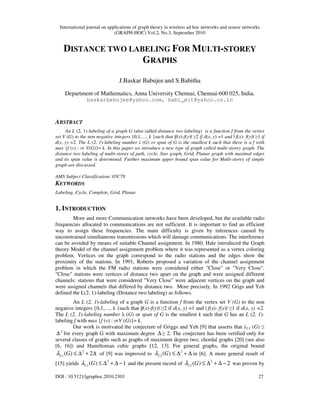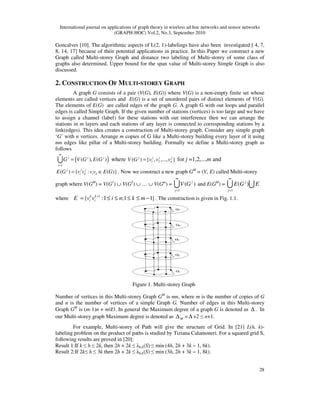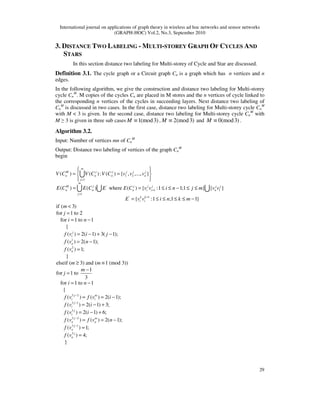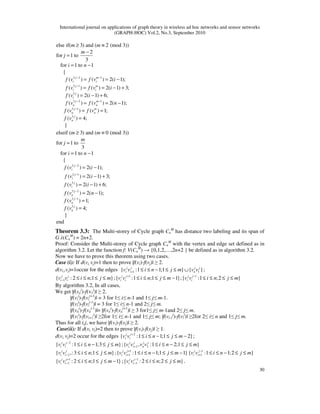Anzeige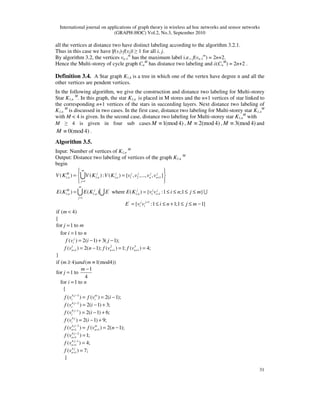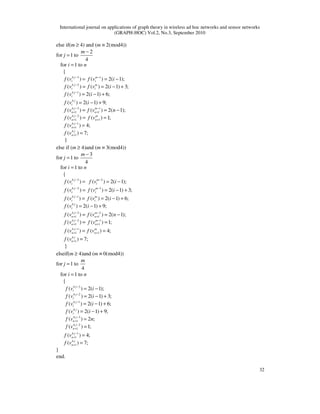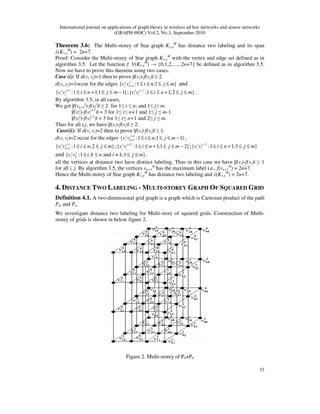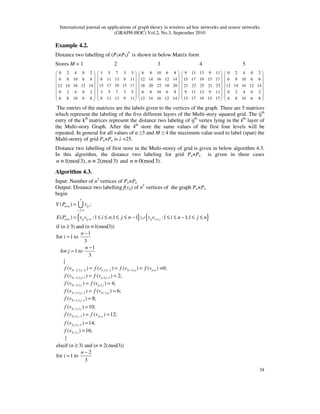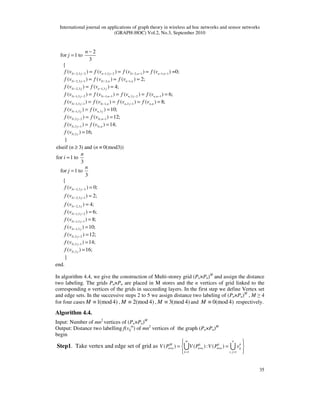Anzeige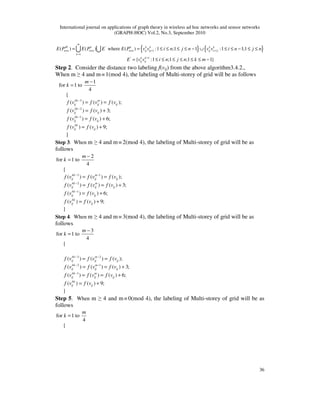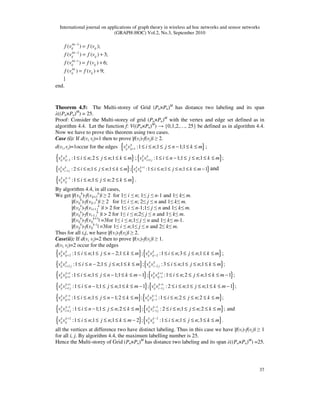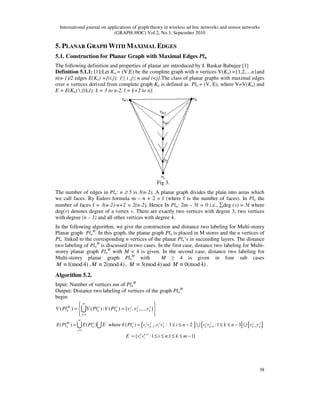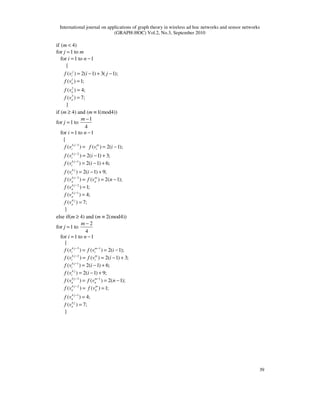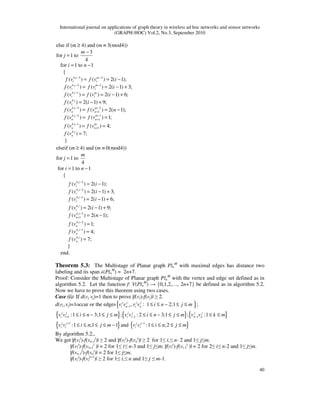Anzeige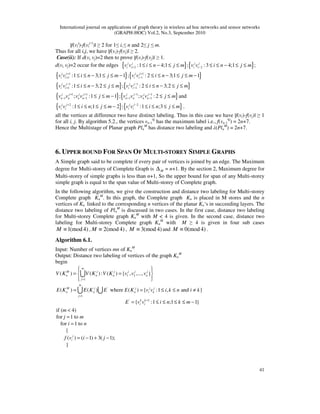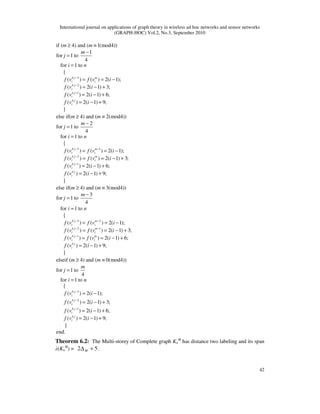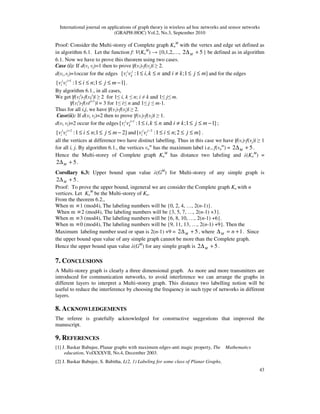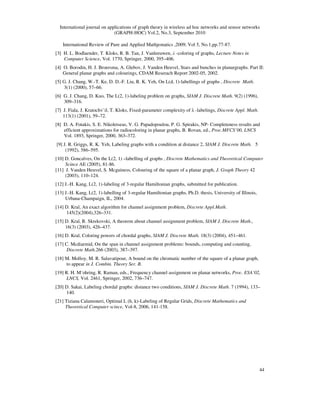Nächste SlideShare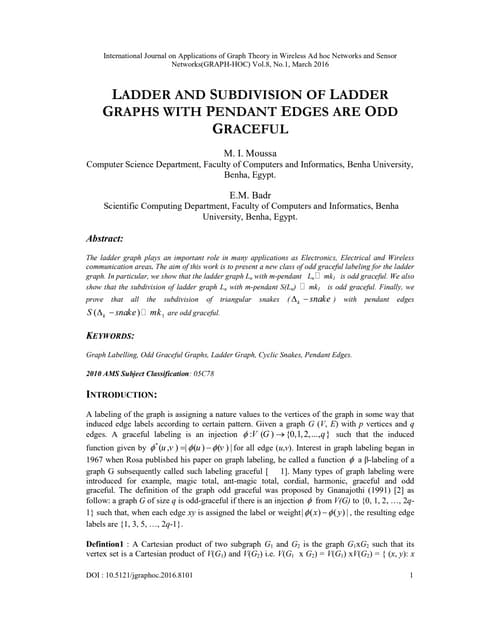LADDER AND SUBDIVISION OF LADDER GRAPHS WITH PENDANT EDGES ARE ODD GRACEFUL
1 von 18
Anzeige

### DISTANCE TWO LABELING FOR MULTI-STOREY GRAPHS

1. International journal on applications of graph theory in wireless ad hoc networks and sensor networks (GRAPH-HOC) Vol.2, No.3, September 2010 DOI : 10.5121/jgraphoc.2010.2303 27 DISTANCE TWO LABELING FOR MULTI-STOREY GRAPHS J.Baskar Babujee and S.Babitha Department of Mathematics, Anna University Chennai, Chennai-600 025, India. baskarbabujee@yahoo.com, babi_mit@yahoo.co.in ABSTRACT An L (2, 1)-labeling of a graph G (also called distance two labeling) is a function f from the vertex set V (G) to the non negative integers {0,1,…, k }such that |f(x)-f(y)| ≥2 if d(x, y) =1 and | f(x)- f(y)| ≥1 if d(x, y) =2. The L (2, 1)-labeling number λ (G) or span of G is the smallest k such that there is a f with max {f (v) : vє V(G)}= k. In this paper we introduce a new type of graph called multi-storey graph. The distance two labeling of multi-storey of path, cycle, Star graph, Grid, Planar graph with maximal edges and its span value is determined. Further maximum upper bound span value for Multi-storey of simple graph are discussed. AMS Subject Classification: 05C78 KEYWORDS Labeling, Cycle, Complete, Grid, Planar 1. INTRODUCTION More and more Communication networks have been developed, but the available radio frequencies allocated to communications are not sufficient. It is important to find an efficient way to assign these frequencies. The main difficulty is given by inferences caused by unconstrained simultaneous transmissions which will damage communications. The interference can be avoided by means of suitable Channel assignment. In 1980, Hale introduced the Graph theory Model of the channel assignment problem where it was represented as a vertex coloring problem. Vertices on the graph correspond to the radio stations and the edges show the proximity of the stations. In 1991, Roberts proposed a variation of the channel assignment problem in which the FM radio stations were considered either "Close" or "Very Close". "Close" stations were vertices of distance two apart on the graph and were assigned different channels; stations that were considered "Very Close" were adjacent vertices on the graph and were assigned channels that differed by distance two. More precisely, In 1992 Grigs and Yeh defined the L(2, 1)-labeling (Distance two labeling) as follows. An L (2, 1)-labeling of a graph G is a function f from the vertex set V (G) to the non negative integers {0,1,…, k }such that |f(x)-f(y)| ≥2 if d(x, y) =1 and | f(x)- f(y)| ≥1 if d(x, y) =2. The L (2, 1)-labeling number λ (G) or span of G is the smallest k such that G has an L (2, 1)- labeling f with max {f (v) : vєV (G)}= k. Our work is motivated the conjecture of Griggs and Yeh  that asserts that λ2,1 (G) ≤ ∆ 2 for every graph G with maximum degree ∆ ≥ 2. The conjecture has been verified only for several classes of graphs such as graphs of maximum degree two, chordal graphs  (see also [6, 16]) and Hamiltonian cubic graphs [12, 13]. For general graphs, the original bound 2 2,1( ) 2Gλ ≤ ∆ + ∆ of  was improved to 2 2,1( )Gλ ≤ ∆ + ∆ in . A more general result of  yields 2 2,1( ) 1Gλ ≤ ∆ + ∆ − and the present record of 2 2,1( ) 2Gλ ≤ ∆ + ∆ − was proven by
2. International journal on applications of graph theory in wireless ad hoc networks and sensor networks (GRAPH-HOC) Vol.2, No.3, September 2010 28 Goncalves . The algorithmic aspects of L(2, 1)-labelings have also been investigated [ 4, 7, 8, 14, 17] because of their potential applications in practice. In this Paper we construct a new Graph called Multi-storey Graph and distance two labeling of Multi-storey of some class of graphs also determined. Upper bound for the span value of Multi-storey Simple Graph is also discussed. 2. CONSTRUCTION OF MULTI-STOREY GRAPH A graph G consists of a pair (V(G), E(G)) where V(G) is a non-empty finite set whose elements are called vertices and E(G) is a set of unordered pairs of distinct elements of V(G). The elements of E(G) are called edges of the graph G. A graph G with out loops and parallel edges is called Simple Graph. If the given number of stations (vertices) is too large and we have to assign a channel (label) for these stations with out interference then we can arrange the stations in m layers and each stations of any layer is connected to corresponding stations by a link(edges). This idea creates a construction of Multi-storey graph. Consider any simple graph ‘G’ with n vertices. Arrange m copies of G like a Multi-storey building every layer of it using mn edges like pillar of a Multi-storey building. Formally we define a Multi-storey graph as follows ( ) 1 ( ), ( ) m j j j i G V G E G = =U where 1 2( ) { , ,..., }j j j j nV G v v v= for j =1,2,...,m and ( ) { : ( )}j j j i k i kE G v v v v E G= ∈ . Now we construct a new graph GM = (V, E) called Multi-storey graph where V(GM ) = V(G1 ) ∪ V(G2 ) ∪ … ∪ V(Gm ) = 1 ( ) m j j V G = U and E(GM ) = ' 1 ( ) m j j E G E = U U where ' 1 { :1 ;1 1}k k i iE v v i n k m+ = ≤ ≤ ≤ ≤ − . The construction is given in Fig. 1.1. Figure 1. Multi-storey Graph Number of vertices in this Multi-storey Graph GM is mn, where m is the number of copies of G and n is the number of vertices of a simple Graph G. Number of edges in this Multi-storey Graph GM is (m-1)n + m|E|. In general the Maximum degree of a graph G is denoted as ∆ . In our Multi-storey graph Maximum degree is denoted as M∆ = ∆ +2 ≤ n+1. For example, Multi-storey of Path will give the structure of Grid. In  L(h, k)- labeling problem on the product of paths is studied by Tiziana Calamoneri. For a squared grid S, following results are proved in : Result 1:If k ≤ h ≤ 2k, then 2h + 2k ≤ λh,k(S) ≤ min (4h, 2h + 3k − 1, 6k). Result 2:If 2k≤ h ≤ 3k then 2h + 2k ≤ λh,k(S) ≤ min (3h, 2h + 3k − 1, 8k).
3. International journal on applications of graph theory in wireless ad hoc networks and sensor networks (GRAPH-HOC) Vol.2, No.3, September 2010 29 3. DISTANCE TWO LABELING - MULTI-STOREY GRAPH OF CYCLES AND STARS In this section distance two labeling for Multi-storey of Cycle and Star are discussed. Definition 3.1. The cycle graph or a Circuit graph Cn is a graph which has n vertices and n edges. In the following algorithm, we give the construction and distance two labeling for Multi-storey cycle Cn M . M copies of the cycles Cn are placed in M stores and the n vertices of cycle linked to the corresponding n vertices of the cycles in succeeding layers. Next distance two labeling of Cn M is discussed in two cases. In the first case, distance two labeling for Multi-storey cycle Cn M with M < 3 is given. In the second case, distance two labeling for Multi-storey cycle Cn M with M ≥ 3 is given in three sub cases 1(mod3)M ≡ , 2(mod3)M ≡ and 0(mod3)M ≡ . Algorithm 3.2. Input: Number of vertices mn of Cn M Output: Distance two labeling of vertices of the graph Cn M begin 1 2 1 ' 1 1 1 ' 1 ( ) ( ): ( ) { , ,..., } ( ) ( ) where ( ) { :1 1;1 } { } { :1 ;1 1} m M j j j j j n n n n j m M j j j j j j n n n i i n j k k i i V C V C V C v v v E C E C E E C v v i n j m v v E v v i n k m = + = +    = =     = = ≤ ≤ − ≤ ≤ = ≤ ≤ ≤ ≤ − U U U U 1 2 if ( 3) for 1 to 2 for 1 to 1 { ( ) 2( 1) 3( 1); ( ) 2( 1); ( ) 1; } elseif ( 3) and ( 1 (mod 3)) 1 for 1 to 3 for 1 to 1 { ( j i n n i m j i n f v i j f v n f v m m m j i n f v < = = − = − + − = − = ≥ ≡ − = = − 3 2 3 1 3 3 2 3 1 3 ) ( ) 2( 1); ( ) 2( 1) 3; ( ) 2( 1) 6; ( ) ( ) 2( 1); ( ) 1; ( ) 4; } j m i j i j i j m n n j n j n f v i f v i f v i f v f v n f v f v − − − − = = − = − + = − + = = − = =
4. International journal on applications of graph theory in wireless ad hoc networks and sensor networks (GRAPH-HOC) Vol.2, No.3, September 2010 30 3 2 1 else if( 3) and ( 2 (mod 3)) 2 for 1 to 3 for 1 to 1 { ( ) ( ) 2( 1);j m i i m m m j i n f v f v i− − ≥ ≡ − = = − = = − 3 1 3 3 2 1 3 1 3 ( ) ( ) 2( 1) 3; ( ) 2( 1) 6; ( ) ( ) 2( 1); ( ) ( ) 1; ( ) 4; } j m i i j i j m n n j m n n j n f v f v i f v i f v f v n f v f v f v − − − − = = − + = − + = = − = = = elseif ( 3) and ( 0 (mod 3)) for 1 to 3 m m m j ≥ ≡ = 3 2 for 1 to 1 { ( ) 2( 1);j i i n f v i− = − = − 3 1 ( ) 2( 1) 3;j if v i− = − + 3 ( ) 2( 1) 6;j if v i= − + 3 2 3 1 3 ( ) 2( 1); ( ) 1; ( ) 4; } end j n j n j n f v n f v f v − − = − = = Theorem 3.3: The Multi-storey of Cycle graph Cn M has distance two labeling and its span of G λ(Cn M ) = 2n+2. Proof: Consider the Multi-storey of Cycle graph Cn M with the vertex and edge set defined as in algorithm 3.2. Let the function f: V(Cn M ) → {0,1,2,…,2n+2 } be defined as in algorithm 3.2. Now we have to prove this theorem using two cases. Case (i): If d(vi, vj)=1 then to prove |f(vi)-f(vj)| ≥ 2. d(vi,,vj)=1occur for the edges 1 1{ :1 1;1 } { }j j j j i i nv v i n j m v v+ ≤ ≤ − ≤ ≤ ∪ ; 1{ : 2 ;1 }j j i iv v i n j m− ≤ ≤ ≤ ≤ ; 1 { :1 ;1 1}j j i iv v i n j m+ ≤ ≤ ≤ ≤ − ; 1 { :1 ;2 }j j i iv v i n j m− ≤ ≤ ≤ ≤ By algorithm 3.2, In all cases, We get |f(vn j )-f(v1 j )| ≥ 2. |f(vi j )-f(vi j+1 )| = 3 for 1≤ i≤ n-1 and 1≤ j≤ m-1. |f(vi j )-f(vi j-1 )| = 3 for 1≤ i≤ n-1 and 2≤ j≤ m. |f(vn j )-f(vn j-1 )|= |f(vn j )-f(vn j+1 )| ≥ 3 for1≤ j≤ m-1and 2≤ j≤ m. |f(vi j )-f(vi+1 j )| ≥2for 1≤ i≤ n-1 and 1≤ j≤ m; |f(vi-1 j )-f(vi j )| ≥2for 2≤ i≤ n and 1≤ j≤ m. Thus for all i,j, we have |f(vi)-f(vj)| ≥ 2. Case(ii): If d(vi, vj)=2 then to prove |f(vi)-f(vj)| ≥ 1. d(vi, vj)=2 occur for the edges 2 { :1 1;1 2}j j i iv v i n j m+ ≤ ≤ − ≤ ≤ − ; 2 { :1 1;3 }j j i iv v i n j m− ≤ ≤ − ≤ ≤ ; 2 2{ , :1 2;1 }j j j j i i nv v v v i n j m+ ≤ ≤ − ≤ ≤ 2{ ,:3 ;1 }j j i iv v i n j m− ≤ ≤ ≤ ≤ ; 1 1{ :1 1;1 1}j j i iv v i n j m+ + ≤ ≤ − ≤ ≤ − 1 1{ :1 1;2 }j j i iv v i n j m− + ≤ ≤ − ≤ ≤ 1 1{ : 2 ;1 1}j j i iv v i n j m+ − ≤ ≤ ≤ ≤ − ; 1 1{ : 2 ;2 }j j i iv v i n j m− − ≤ ≤ ≤ ≤ .
5. International journal on applications of graph theory in wireless ad hoc networks and sensor networks (GRAPH-HOC) Vol.2, No.3, September 2010 31 all the vertices at distance two have distinct labeling according to the algorithm 3.2.1. Thus in this case we have |f(vi)-f(vj)| ≥ 1 for all i, j. By algorithm 3.2, the vertices vn-1 m has the maximum label i.e., f(vn-1 m ) = 2n+2. Hence the Multi-storey of cycle graph Cn M has distance two labeling and λ(Cn M ) = 2n+2 . Definition 3.4. A Star graph K1,k is a tree in which one of the vertex have degree n and all the other vertices are pendent vertices. In the following algorithm, we give the construction and distance two labeling for Multi-storey Star K1,n M . In this graph, the star K1,n is placed in M stores and the n+1 vertices of star linked to the corresponding n+1 vertices of the stars in succeeding layers. Next distance two labeling of K1,n M is discussed in two cases. In the first case, distance two labeling for Multi-storey star K1,n M with M < 4 is given. In the second case, distance two labeling for Multi-storey star K1,n M with M ≥ 4 is given in four sub cases 1(mod 4)M ≡ , 2(mod 4)M ≡ , 3(mod 4)M ≡ and 0(mod 4)M ≡ . Algorithm 3.5. Input: Number of vertices of K1,n M Output: Distance two labeling of vertices of the graph K1,n M begin 1, 1, 1, 1 2 1 1 ( ) ( ): ( ) { , ,..., , } m M j j j j j j n n n n n j V K V K V K v v v v + =    = =     U ' 1, 1, 1, 1 1 1 ' 1 ( ) ( ) where ( ) { :1 ;1 } { :1 1;1 1} m M j j j j n n n i j j j i i E K E K E E K v v i n j m E v v i n j m + = + = = ≤ ≤ ≤ ≤ = ≤ ≤ + ≤ ≤ − UU U if ( 4) { for 1 to m j m < = 1 2 3 1 1 1 for 1 to ( ) 2( 1) 3( 1); ( ) 2( 1); ( ) 1; ( ) 4; } j i n n n i n f v i j f v n f v f v+ + + = = − + − = − = = if ( 4) ( 1(mod4)) 1 for 1 to 4 for 1 to { m and m m j i n ≥ ≡ − = = 4 3 4 2 4 1 4 4 3 1 1 4 2 1 4 1 1 4 1 ( ) ( ) 2( 1); ( ) 2( 1) 3; ( ) 2( 1) 6; ( ) 2( 1) 9; ( ) ( ) 2( 1); ( ) 1; ( ) 4; ( ) 7; j m i i j i j i j i j m n n j n j n j n f v f v i f v i f v i f v i f v f v n f v f v f v − − − − + + − + − + + = = − = − + = − + = − + = = − = = = }
6. International journal on applications of graph theory in wireless ad hoc networks and sensor networks (GRAPH-HOC) Vol.2, No.3, September 2010 32 4 3 1 4 2 4 1 else if( 4) and ( 2(mod4)) 2 for 1 to 4 for 1 to { ( ) ( ) 2( 1); ( ) ( ) 2( 1) 3; ( ) 2( 1) 6; j m i i j m i i j i m m m j i n f v f v i f v f v i f v i − − − − ≥ ≡ − = = = = − = = − + = − + 4 4 3 1 1 1 4 2 1 1 4 1 1 4 1 ( ) 2( 1) 9; ( ) ( ) 2( 1); ( ) ( ) 1; ( ) 4; ( ) 7; } j i j m n n j m n n j n j n f v i f v f v n f v f v f v f v − − + + − + + − + + = − + = = − = = = = 4 3 2 else if ( 4)and ( 3(mod4)) 3 for 1 to 4 for 1 to { ( ) ( ) 2( 1);j m i i m m m j i n f v f v i− − ≥ ≡ − = = = = − 4 2 1 ( ) ( ) 2( 1) 3;j m i if v f v i− − = = − + 4 1 4 ( ) ( ) 2( 1) 6; ( ) 2( 1) 9; j m i i j i f v f v i f v i − = = − + = − + 4 3 2 1 1 4 2 1 1 1 ( ) ( ) 2( 1); ( ) ( ) 1; j m n n j m n n f v f v n f v f v − − + + − − + + = = − = = 4 1 1 1( ) ( ) 4;j m n nf v f v− + += = 4 1( ) 7; } j nf v + = elseif( 4)and ( 0(mod4)) for 1 to 4 m m m j ≥ ≡ = 4 3 4 2 4 1 4 4 3 1 4 2 1 for 1 to { ( ) 2( 1); ( ) 2( 1) 3; ( ) 2( 1) 6; ( ) 2( 1) 9; ( ) 2 ; ( ) 1; j i j i j i j i j n j n i n f v i f v i f v i f v i f v n f v − − − − + − + = = − = − + = − + = − + = = 4 1 1 4 1 ( ) 4; ( ) 7; } end. j n j n f v f v − + + = =
7. International journal on applications of graph theory in wireless ad hoc networks and sensor networks (GRAPH-HOC) Vol.2, No.3, September 2010 33 Theorem 3.6: The Multi-storey of Star graph K1,n M has distance two labeling and its span λ(K1,n M ) = 2n+7. Proof: Consider the Multi-storey of Star graph K1,n M with the vertex and edge set defined as in algorithm 3.5. Let the function f: V(K1,n M ) → {0,1,2,…, 2n+7} be defined as in algorithm 3.5. Now we have to prove this theorem using two cases. Case (i): If d(vi, vj)=1 then to prove |f(vi)-f(vj)| ≥ 2. d(vi,,vj)=1occur for the edges 1{ :1 ;1 }j j i nv v i n j m+ ≤ ≤ ≤ ≤ and 1 { :1 1;1 1}j j i iv v i n j m+ ≤ ≤ + ≤ ≤ − ; 1 { :1 1;2 }j j i iv v i n j m− ≤ ≤ + ≤ ≤ . By algorithm 3.5, in all cases, We get |f(vn+1 j )-f(vi j )| ≥ 2 for 1≤ i ≤ n; and 1≤ j≤ m. |f(vi j )-f(vi j+1 )| = 3 for 1≤ i≤ n+1 and 1≤ j ≤ m-1. |f(vi j )-f(vi j-1 )| = 3 for 1≤ i≤ n+1 and 2≤ j ≤ m. Thus for all i,j, we have |f(vi)-f(vj)| ≥ 2. Case(ii): If d(vi, vj)=2 then to prove |f(vi)-f(vj)| ≥ 1. d(vi, vj)=2 occur for the edges 1 1{ :1 ;1 1}j j i nv v i n j m+ + ≤ ≤ ≤ ≤ − , 1 1{ :1 ;2 }j j i nv v i n j m− + ≤ ≤ ≤ ≤ ; 2 { :1 1;1 2}j j i iv v i n j m+ ≤ ≤ + ≤ ≤ − ; 2 { :1 1;3 }j j i iv v i n j m− ≤ ≤ + ≤ ≤ and { :1 , and ;1 }j j i kv v i k n i k j m≤ ≤ ≠ ≤ ≤ . all the vertices at distance two have distinct labeling. Thus in this case we have |f(vi)-f(vj)| ≥ 1 for all i, j. By algorithm 3.5, the vertices vn+1 4j has the maximum label i.e., f(vn+1 4j ) = 2n+7. Hence the Multi-storey of Star graph K1,n M has distance two labeling and λ(K1,n M ) = 2n+7. 4. DISTANCE TWO LABELING - MULTI-STOREY GRAPH OF SQUARED GRID Definition 4.1. A two-dimensional grid graph is a graph which is Cartesian product of the path Pm and Pn. We investigate distance two labeling for Multi-story of squared grids. Construction of Multi- storey of grids is shown in below figure 2. Figure 2. Multi-storey of P4×P4
8. International journal on applications of graph theory in wireless ad hoc networks and sensor networks (GRAPH-HOC) Vol.2, No.3, September 2010 34 Example 4.2. Distance two labelling of (P5×P5)5 is shown in below Matrix form Stores M = 1 2 3 4 5 0 2 4 0 2 3 5 7 3 5 6 8 10 6 8 9 11 13 9 11 6 8 10 6 8 9 11 13 9 11 12 14 16 12 14 15 17 19 15 17 12 14 16 12 14 15 17 19 15 17 18 20 22 18 20 21 23 25 21 23 0 2 4 0 2 3 5 7 3 5 6 8 10 6 8 9 11 13 9 11 6 8 10 6 8 9 11 13 9 11 12 14 16 12 14 15 1                                                 0 2 4 0 2 6 8 10 6 8 12 14 16 12 14 0 2 4 0 2 7 19 15 17 6 8 10 6 8                                 The entries of the matrices are the labels given to the vertices of the graph. There are 5 matrices which represent the labeling of the five different layers of the Multi-story squared grid. The ijth entry of the kth matrices represent the distance two labelng of ijth vertex lying in the kth layer of the Multi-story Graph. After the 4th store the same values of the first four levels will be repeated. In general for all values of n ≥3 and M ≥ 4 the maximum value used to label (span) the Multi-storey of grid Pn×Pn is λ =25. Distance two labelling of first store in the Multi-storey of grid is given in below algorithm 4.3. In this algorithm, the distance two labeling for grid Pn×Pn is given in three cases 1(mod3)n ≡ , 2(mod3)n ≡ and 0(mod3)n ≡ . Algorithm 4.3. Input: Number of n2 vertices of Pn×Pn Output: Distance two labelling f(vij) of n2 vertices of the graph Pn×Pn begin }{ { } , 1 1 1 ( ) ; ( ) :1 ;1 1 :1 1;1 n n n ij i j n n ij ij ij i j V P v E P v v i n j n v v i n j n × = × + + = = ≤ ≤ ≤ ≤ − ∪ ≤ ≤ − ≤ ≤ U if ( 3) and ( 1(mod3)) 1 for 1 to 3 n n n i ≥ ≡ − = 1 for 1 to 3 n j − = 3 2,3 2 ,3 2 3 2, , 3 2,3 1 ,3 1 3 2,3 ,3 3 1,3 2 3 1, 3 1,3 1 { ( ) ( ) ( ) ( ) =0; ( ) ( ) 2; ( ) ( ) 4; ( ) ( ) 6; ( ) 8; i j n j i n n n i j n j i j n j i j i n i j f v f v f v f v f v f v f v f v f v f v f v − − − − − − − − − − − − − = = = = = = = = = = 3 1,3 3 ,3 2 3 , ( ) 10; ( ) ( ) 12; i j i j i n f v f v f v − − = = = 3 ,3 1 3 ,3 ( ) 14; ( ) 16; } i j i j f v f v − = = elseif ( 3) and ( 2(mod3)) 2 for 1 to 3 n n n i ≥ ≡ − =
9. International journal on applications of graph theory in wireless ad hoc networks and sensor networks (GRAPH-HOC) Vol.2, No.3, September 2010 35 3 2,3 2 1,3 2 3 2, 1 1, 1 3 2,3 1 3 2, 1, 3 2,3 1,3 3 1,3 2 3 1, 1 2 for 1 to 3 { ( ) ( ) ( ) ( ) =0; ( ) ( ) ( ) 2; ( ) ( ) 4; ( ) ( ) ( i j n j i n n n i j i n n n i j n j i j i n n j f v f v f v f v f v f v f v f v f v f v f v f − − − − − − − − − − − − − − − − − − − = = = = = = = = = = = ,3 2 , 1 3 1,3 1 3 1, ,3 1 , 3 1,3 ,3 3 ,3 2 3 , 1 3 ,3 1 3 , 3 ,3 ) ( ) 6; ( ) ( ) ( ) ( ) 8; ( ) ( ) 10; ( ) ( ) 12; ( ) ( ) 14; ( ) 16; } n j n n i j i n n j n n i j n j i j i n i j i n i j v f v f v f v f v f v f v f v f v f v f v f v f v − − − − − − − − − − = = = = = = = = = = = = = 3 2,3 2 elseif ( 3) and ( 0(mod3)) for 1 to 3 for 1 to 3 { ( ) 0;i j n n n i n j f v − − ≥ ≡ = = = 3 2,3 1( ) 2;i jf v − − = 3 2,3 3 1,3 2 3 1,3 1 3 1,3 3 ,3 2 3 ,3 1 3 ,3 ( ) 4; ( ) 6; ( ) 8; ( ) 10; ( ) 12; ( ) 14; ( ) 16; } i j i j i j i j i j i j i j f v f v f v f v f v f v f v − − − − − − − − = = = = = = = end. In algorithm 4.4, we give the construction of Multi-storey grid (Pn×Pn)M and assign the distance two labeling. The grids Pn×Pn are placed in M stores and the n vertices of grid linked to the corresponding n vertices of the grids in succeeding layers. In the first step we define Vertex set and edge sets. In the successive steps 2 to 5 we assign distance two labeling of (Pn×Pn)M , M ≥ 4 for four cases 1(mod 4)M ≡ , 2(mod 4)M ≡ , 3(mod 4)M ≡ and 0(mod 4)M ≡ respectively. Algorithm 4.4. Input: Number of mn2 vertices of (Pn×Pn)M Output: Distance two labelling f(vij m ) of mn2 vertices of the graph (Pn×Pn)M begin Step1. Take vertex and edge set of grid as 1 , 1 ( ) ( ): ( ) m n M k k k n n n n n n ij k i j V P V P V P v× × × = =    = =     U U
10. International journal on applications of graph theory in wireless ad hoc networks and sensor networks (GRAPH-HOC) Vol.2, No.3, September 2010 36 }{ { }' 1 1 1 ' 1 ( ) ( ) where ( ) :1 ;1 1 :1 1;1 { :1 ;1 ;1 1} m M k k k k k k n n n n n n ij ij ij i j k k k ij ij E P E P E E P v v i n j n v v i n j n E v v i n j n k m × × × + + = + = = ≤ ≤ ≤ ≤ − ∪ ≤ ≤ − ≤ ≤ = ≤ ≤ ≤ ≤ ≤ ≤ − U U Step 2. Consider the distance two labeling f(vij) from the above algorithm3.4.2., When m ≥ 4 and m ≡ 1(mod 4), the labeling of Multi-storey of grid will be as follows 4 3 4 2 4 1 4 1 for 1 to 4 { ( ) ( ) ( ); ( ) ( ) 3; ( ) ( ) 6; ( ) ( ) 9; } k m ij ij ij k ij ij k ij ij k ij ij m k f v f v f v f v f v f v f v f v f v − − − − = = = = + = + = + Step 3. When m ≥ 4 and m ≡ 2(mod 4), the labeling of Multi-storey of grid will be as follows 4 3 1 4 2 4 1 4 2 for 1 to 4 { ( ) ( ) ( ); ( ) ( ) ( ) 3; ( ) ( ) 6; ( ) ( ) 9; } k m ij ij ij k m ij ij ij k ij ij k ij ij m k f v f v f v f v f v f v f v f v f v f v − − − − − = = = = = + = + = + Step 4. When m ≥ 4 and m ≡ 3(mod 4), the labeling of Multi-storey of grid will be as follows 3 for 1 to 4 { m k − = 4 3 2 4 2 1 4 1 4 ( ) ( ) ( ); ( ) ( ) ( ) 3; ( ) ( ) ( ) 6; ( ) ( ) 9; } k m ij ij ij k m ij ij ij k m ij ij ij k ij ij f v f v f v f v f v f v f v f v f v f v f v − − − − − = = = = + = = + = + Step 5. When m ≥ 4 and m ≡ 0(mod 4), the labeling of Multi-storey of grid will be as follows for 1 to 4 { m k =
11. International journal on applications of graph theory in wireless ad hoc networks and sensor networks (GRAPH-HOC) Vol.2, No.3, September 2010 37 4 3 4 2 4 1 4 ( ) ( ); ( ) ( ) 3; ( ) ( ) 6; ( ) ( ) 9; } k ij ij k ij ij k ij ij k ij ij f v f v f v f v f v f v f v f v − − − = = + = + = + end. Theorem 4.5: The Multi-storey of Grid (Pn×Pn)M has distance two labeling and its span λ((Pn×Pn)M ) = 25. Proof: Consider the Multi-storey of grid (Pn×Pn)M with the vertex and edge set defined as in algorithm 4.4. Let the function f: V((Pn×Pn)M ) → {0,1,2,…, 25} be defined as in algorithm 4.4. Now we have to prove this theorem using two cases. Case (i): If d(vi, vj)=1 then to prove |f(vi)-f(vj)| ≥ 2. d(vi,,vj)=1occur for the edges }{ 1 :1 ;1 1;1k k ij ijv v i n j n k m+ ≤ ≤ ≤ ≤ − ≤ ≤ ; }{ 1 :1 ;2 ;1k k ij ijv v i n j n k m− ≤ ≤ ≤ ≤ ≤ ≤ ;{ }1 :1 1;1 ;1 ;k k ij i jv v i n j n k m+ ≤ ≤ − ≤ ≤ ≤ ≤ { } }{ 1 1 :2 ;1 ;1 ; :1 ;1 ;1 1k k k k ij i j ij ijv v i n j n k m v v i n j n k m+ − ≤ ≤ ≤ ≤ ≤ ≤ ≤ ≤ ≤ ≤ ≤ ≤ − and }{ 1 :1 ;1 ;2k k ij ijv v i n j n k m− ≤ ≤ ≤ ≤ ≤ ≤ . By algorithm 4.4, in all cases, We get |f(vij k )-f(vij+1 k )| ≥ 2 for 1≤ i ≤ n; 1≤ j ≤ n-1 and 1≤ k≤ m. |f(vij k )-f(vij-1 k )| ≥ 2 for 1≤ i ≤ n; 2≤ j ≤ n and 1≤ k≤ m. |f(vij k )-f(vi+1 j k )| > 2 for 1≤ i ≤ n-1;1≤ j ≤ n and 1≤ k≤ m. |f(vij k )-f(vi-1 j k )| > 2 for 1≤ i ≤ n;2≤ j ≤ n and 1≤ k≤ m. |f(vij k )-f(vij k+1 | =3for 1≤ i ≤ n;1≤ j ≤ n and 1≤ k≤ m-1. |f(vij k )-f(vij k-1 | =3for 1≤ i ≤ n;1≤ j ≤ n and 2≤ k≤ m. Thus for all i,j, we have |f(vi)-f(vj)| ≥ 2. Case(ii): If d(vi, vj)=2 then to prove |f(vi)-f(vj)| ≥ 1. d(vi, vj)=2 occur for the edges }{ }{2 2:1 ;1 2;1 ; :1 ;3 ;1k k k k ij ij ij ijv v i n j n k m v v i n j n k m+ −≤ ≤ ≤ ≤ − ≤ ≤ ≤ ≤ ≤ ≤ ≤ ≤ ; { } { }2 2:1 2;1 ;1 ; :3 ;1 ;1 ;k k k k ij i j ij i jv v i n j n k m v v i n j n k m+ −≤ ≤ − ≤ ≤ ≤ ≤ ≤ ≤ ≤ ≤ ≤ ≤ }{ }{1 1 1 1:1 ;1 1;1 1 ; :1 ;2 ;1 1 ;k k k k ij ij ij ijv v i n j n k m v v i n j n k m+ + + −≤ ≤ ≤ ≤ − ≤ ≤ − ≤ ≤ ≤ ≤ ≤ ≤ − }{ }{1 1 1 1:1 1;1 ;1 1 ; : 2 ;1 ;1 1 ;k k k k ij i j ij i jv v i n j n k m v v i n j n k m+ + + −≤ ≤ − ≤ ≤ ≤ ≤ − ≤ ≤ ≤ ≤ ≤ ≤ − }{ }{1 1 1 1:1 ;1 1;2 ; :1 ;2 ;2 ;k k k k ij ij ij ijv v i n j n k m v v i n j n k m− − + −≤ ≤ ≤ ≤ − ≤ ≤ ≤ ≤ ≤ ≤ ≤ ≤ }{ }{1 1 1 1:1 1;1 ;2 ; : 2 ;1 ;2 ; andk k k k ij i j ij i jv v i n j n k m v v i n j n k m− − + −≤ ≤ − ≤ ≤ ≤ ≤ ≤ ≤ ≤ ≤ ≤ ≤ }{ }{2 2 :1 ;1 ;1 2 ; :1 ;1 ;3 .k k k k ij ij ij ijv v i n j n k m v v i n j n k m+ − ≤ ≤ ≤ ≤ ≤ ≤ − ≤ ≤ ≤ ≤ ≤ ≤ all the vertices at difference two have distinct labeling. Thus in this case we have |f(vi)-f(vj)| ≥ 1 for all i, j. By algorithm 4.4, the maximum labelling number is 25. Hence the Multi-storey of Grid (Pn×Pn)M has distance two labeling and its span λ((Pn×Pn)M ) =25.
12. International journal on applications of graph theory in wireless ad hoc networks and sensor networks (GRAPH-HOC) Vol.2, No.3, September 2010 38 5. PLANAR GRAPH WITH MAXIMAL EDGES 5.1. Construction for Planar Graph with Maximal Edges Pln The following definition and properties of planar are introduced by J. Baskar Babujee  Definition 5.1.1: :Let Kn = (V,E) be the complete graph with n vertices V(Kn) ={1,2,…n}and n(n-1)/2 edges E(Kn) ={(i.j): 1≤ i ,j≤ n and i<j}.The class of planar graphs with maximal edges over n vertices derived from complete graph Kn is defined as Pln = (V, E), where V=V(Kn) and E = E(Kn) {(k,l): k = 3 to n-2, l = k+2 to n}. Fig 3. The number of edges in Pln: n ≥ 5 is 3(n-2). A planar graph divides the plain into areas which we call faces. By Eulers formula m – n + 2 = f (where f is the number of faces). In Pln the number of faces f = 3(n-2)-n+2 = 2(n-2). Hence In Pln: 2m – 3f = 0 i.e., ∑deg (v) = 3f where deg(v) denotes degree of a vertex v. There are exactly two vertices with degree 3, two vertices with degree (n – 1) and all other vertices with degree 4. In the following algorithm, we give the construction and distance two labeling for Multi-storey Planar graph Pln M . In this graph, the planar graph Pln is placed in M stores and the n vertices of Pln linked to the corresponding n vertices of the planar Pln‘s in succeeding layers. The distance two labeling of Pln M is discussed in two cases. In the first case, distance two labeling for Multi- storey planar graph Pln M with M < 4 is given. In the second case, distance two labeling for Multi-storey planar graph Pln M with M ≥ 4 is given in four sub cases 1(mod 4)M ≡ , 2(mod 4)M ≡ , 3(mod 4)M ≡ and 0(mod 4)M ≡ . Algorithm 5.2. Input: Number of vertices mn of Pln M Output: Distance two labeling of vertices of the graph Pln M begin 1 2 1 ( ) ( ): ( ) { , ,..., } m M j j j j j n n n n j V Pl V Pl V Pl v v v =    = =     U { } { } { }' 1 1 1 1 ' 1 ( ) ( ) where ( ) , : 1 2 :1 3 { :1 ;1 1} m M j j j j j j j j j j n n n i n i n k k n n j k k i i E Pl E Pl E E Pl v v v v i n v v k n v v E v v i n k m − + − = + = = ≤ ≤ − ≤ ≤ − = ≤ ≤ ≤ ≤ − U UU U
13. International journal on applications of graph theory in wireless ad hoc networks and sensor networks (GRAPH-HOC) Vol.2, No.3, September 2010 39 if ( 4) for 1 to for 1 to 1 { m j m i n < = = − 1 ( ) 2( 1) 3( 1); ( ) 1; j i n f v i j f v = − + − = 2 3 ( ) 4; ( ) 7; } n n f v f v = = if ( 4) and ( 1(mod4)) 1 for 1 to 4 m m m j ≥ ≡ − = 4 3 for 1 to 1 { ( ) ( ) 2( 1);j m i i i n f v f v i− = − = = − 4 2 4 1 ( ) 2( 1) 3; ( ) 2( 1) 6; j i j i f v i f v i − − = − + = − + 4 4 3 4 2 4 1 4 ( ) 2( 1) 9; ( ) ( ) 2( 1); ( ) 1; ( ) 4; ( ) 7; } j i j m n n j n j n j n f v i f v f v n f v f v f v − − − = − + = = − = = = else if( 4) and ( 2(mod4)) 2 for 1 to 4 for 1 to 1 m m m j i n ≥ ≡ − = = − 4 3 1 4 2 4 1 4 4 3 1 4 2 { ( ) ( ) 2( 1); ( ) ( ) 2( 1) 3; ( ) 2( 1) 6; ( ) 2( 1) 9; ( ) ( ) 2( 1); ( ) ( ) 1; j m i i j m i i j i j i j m n n j m n n f v f v i f v f v i f v i f v i f v f v n f v f v − − − − − − − = = − = = − + = − + = − + = = − = = 4 1 4 ( ) 4; ( ) 7; } j n j n f v f v − = =
14. International journal on applications of graph theory in wireless ad hoc networks and sensor networks (GRAPH-HOC) Vol.2, No.3, September 2010 40 4 3 2 4 2 1 4 1 4 else if ( 4) and ( 3(mod4)) 3 for 1 to 4 for 1 to 1 { ( ) ( ) 2( 1); ( ) ( ) 2( 1) 3; ( ) ( ) 2( 1) 6; ( ) 2( 1) 9; j m i i j m i i j m i i j i m m m j i n f v f v i f v f v i f v f v i f v i − − − − − ≥ ≡ − = = − = = − = = − + = = − + = − + 4 3 2 1 4 2 1 1 ( ) ( ) 2( 1); ( ) ( ) 1; j m n n j m n n f v f v n f v f v − − + − − + = = − = = 4 1 1 4 ( ) ( ) 4; ( ) 7; } j m n n j n f v f v f v − += = = elseif ( 4) and ( 0(mod4)) for 1 to 4 m m m j ≥ ≡ = for 1 to 1 { i n= − 4 3 4 2 4 1 ( ) 2( 1); ( ) 2( 1) 3; ( ) 2( 1) 6; j i j i j i f v i f v i f v i − − − = − = − + = − + 4 4 3 1 ( ) 2( 1) 9; ( ) 2( 1); j i j n f v i f v n− + = − + = − 4 2 4 1 4 ( ) 1; ( ) 4; ( ) 7; } end. j n j n j n f v f v f v − − = = = Theorem 5.3: The Multistage of Planar graph Pln M with maximal edges has distance two labeling and its span λ(Pln M ) = 2n+7. Proof: Consider the Multistage of Planar graph Pln M with the vertex and edge set defined as in algorithm 5.2. Let the function f: V(Pln M ) → {0,1,2,…, 2n+7} be defined as in algorithm 5.2. Now we have to prove this theorem using two cases. Case (i): If d(vi, vj)=1 then to prove |f(vi)-f(vj)| ≥ 2. d(vi,,vj)=1occur or the edges{ }1, : 1 2;1 ;j j j j i n i nv v v v i n j m− ≤ ≤ − ≤ ≤ { } { } { }1 1 1:1 3;1 ; : 2 3;1 ; :1j j j j j j i i i i n nv v i n j m v v i n j m v v k m+ − −≤ ≤ − ≤ ≤ ≤ ≤ − ≤ ≤ ≤ ≤ { }1 :1 ;1 1j j i iv v i n j m+ ≤ ≤ ≤ ≤ − and { }1 :1 ;2j j i iv v i n j m− ≤ ≤ ≤ ≤ By algorithm 5.2., We get |f(vi j )-f(vn-1 j )| ≥ 2 and |f(vi j )-f(vn j )| ≥ 2 for 1≤ i,≤ n- 2 and 1≤ j≤m. |f(vi j )-f(vi+1 j )| = 2 for 1≤ i≤ n-3 and 1≤ j≤m; |f(vi j )-f(vi-1 j )| = 2 for 2≤ i≤ n-2 and 1≤ j≤m. |f(vn-1 j )-f(vn j )| = 2 for 1≤ j≤m. |f(vi j )-f(vi j+1 )| ≥ 2 for 1≤ i,≤ n and 1≤ j ≤ m-1.
15. International journal on applications of graph theory in wireless ad hoc networks and sensor networks (GRAPH-HOC) Vol.2, No.3, September 2010 41 |f(vi j )-f(vi j-1 )| ≥ 2 for 1≤ i,≤ n and 2≤ j ≤ m. Thus for all i,j, we have |f(vi)-f(vj)| ≥ 2. Case(ii): If d(vi, vj)=2 then to prove |f(vi)-f(vj)| ≥ 1. d(vi, vj)=2 occur for the edges { } { }2 2:1 4;1 ; :3 4;1 ;j j j j i i i iv v i n j m v v i n j m+ −≤ ≤ − ≤ ≤ ≤ ≤ − ≤ ≤ { } { }1 1 1 1:1 3;1 1 ; : 2 3;1 1j j j j i i i iv v i n j m v v i n j m+ + + −≤ ≤ − ≤ ≤ − ≤ ≤ − ≤ ≤ − { } { }1 1 1 1:1 3;2 ; : 2 3;2j j j j i i i iv v i n j m v v i n j m− − + −≤ ≤ − ≤ ≤ ≤ ≤ − ≤ ≤ { } { }1 1 1 1 1 1 1 1; :1 1 ; ; : 2j j j j j j j j n n n n n n n nv v v v j m v v v v j m+ + − − − − − −≤ ≤ − ≤ ≤ and { } { }2 2 :1 ;1 2 ; :1 ;3j j j j i i i iv v i n j m v v i n j m+ − ≤ ≤ ≤ ≤ − ≤ ≤ ≤ ≤ . all the vertices at difference two have distinct labeling. Thus in this case we have |f(vi)-f(vj)| ≥ 1 for all i, j. By algorithm 5.2., the vertices vn-1 4j has the maximum label i.e., f(vn-1 4j ) = 2n+7. Hence the Multistage of Planar graph Pln M has distance two labeling and λ(Pln M ) = 2n+7. 6. UPPER BOUND FOR SPAN OF MULTI-STOREY SIMPLE GRAPHS A Simple graph said to be complete if every pair of vertices is joined by an edge. The Maximum degree for Multi-storey of Complete Graph is M∆ = n+1. By the section 2, Maximum degree for Multi-storey of simple graphs is less than n+1, So the upper bound for span of any Multi-storey simple graph is equal to the span value of Multi-storey of Complete graph. In the following algorithm, we give the construction and distance two labeling for Multi-storey Complete graph Kn M . In this graph, the Complete graph Kn is placed in M stores and the n vertices of Kn linked to the corresponding n vertices of the planar Kn‘s in succeeding layers. The distance two labeling of Pln M is discussed in two cases. In the first case, distance two labeling for Multi-storey Complete graph Kn M with M < 4 is given. In the second case, distance two labeling for Multi-storey Complete graph Kn M with M ≥ 4 is given in four sub cases 1(mod 4)M ≡ , 2(mod 4)M ≡ , 3(mod 4)M ≡ and 0(mod 4)M ≡ . Algorithm 6.1. Input: Number of vertices mn of Kn M Output: Distance two labeling of vertices of the graph Kn M begin 1 2 1 ( ) ( ): ( ) { , ,..., } m M j j j j j n n n n j V K V K V K v v v =    = =     U ' 1 ' 1 ( ) ( ) where ( ) { :1 , and } { :1 ;1 1} m M j j j j n n n i k j k k i i E K E K E E K v v i k n i k E v v i n k m = + = = ≤ ≤ ≠ = ≤ ≤ ≤ ≤ − U U if ( 4) for 1 to for 1 to { ( ) ( 1) 3( 1); } j i m j m i n f v i j < = = = − + −
16. International journal on applications of graph theory in wireless ad hoc networks and sensor networks (GRAPH-HOC) Vol.2, No.3, September 2010 42 4 3 4 2 4 1 4 if ( 4) and ( 1(mod4)) 1 for 1 to 4 for 1 to { ( ) ( ) 2( 1); ( ) 2( 1) 3; ( ) 2( 1) 6; ( ) 2( 1) 9; } j m i i j i j i j i m m m j i n f v f v i f v i f v i f v i − − − ≥ ≡ − = = = = − = − + = − + = − + 4 3 1 4 2 4 1 4 else if( 4) and ( 2(mod4)) 2 for 1 to 4 for 1 to { ( ) ( ) 2( 1); ( ) ( ) 2( 1) 3; ( ) 2( 1) 6; ( ) 2( 1) 9; } j m i i j m i i j i j i m m m j i n f v f v i f v f v i f v i f v i − − − − ≥ ≡ − = = = = − = = − + = − + = − + else if( 4) and ( 3(mod4)) 3 for 1 to 4 m m m j ≥ ≡ − = 4 3 2 4 2 1 4 1 4 for 1 to { ( ) ( ) 2( 1); ( ) ( ) 2( 1) 3; ( ) ( ) 2( 1) 6; ( ) 2( 1) 9; } j m i i j m i i j m i i j i i n f v f v i f v f v i f v f v i f v i − − − − − = = = − = = − + = = − + = − + elseif ( 4) and ( 0(mod4)) for 1 to 4 for 1 to m m m j i n ≥ ≡ = = 4 3 { ( ) 2( 1);j if v i− = − 4 2 ( ) 2( 1) 3;j if v i− = − + 4 1 4 ( ) 2( 1) 6; ( ) 2( 1) 9; } end. j i j i f v i f v i − = − + = − + Theorem 6.2: The Multi-storey of Complete graph Kn M has distance two labeling and its span λ(Kn M ) = 52 +∆M .
17. International journal on applications of graph theory in wireless ad hoc networks and sensor networks (GRAPH-HOC) Vol.2, No.3, September 2010 43 Proof: Consider the Multi-storey of Complete graph Kn M with the vertex and edge set defined as in algorithm 6.1. Let the function f: V(Kn M ) → {0,1,2,…, 52 +∆M } be defined as in algorithm 6.1. Now we have to prove this theorem using two cases. Case (i): If d(vi, vj)=1 then to prove |f(vi)-f(vj)| ≥ 2. d(vi,,vj)=1occur for the edges { :1 , and ;1 }j j i kv v i k n i k j m≤ ≤ ≠ ≤ ≤ and for the edges 1 { :1 ;1 1}j j i iv v i n j m+ ≤ ≤ ≤ ≤ − . By algorithm 6.1., in all cases, We get |f(vi j )-f(vk j )| ≥ 2 for 1≤ i, k ≤ n; i ≠ k and 1≤ j≤ m. |f(vi j )-f(vij+1 )| = 3 for 1≤ i≤ n and 1≤ j ≤ m-1. Thus for all i,j, we have |f(vi)-f(vj)| ≥ 2. Case(ii): If d(vi, vj)=2 then to prove |f(vi)-f(vj)| ≥ 1. d(vi, vj)=2 occur for the edges 1 { :1 , and ;1 1}j j i kv v i k n i k j m+ ≤ ≤ ≠ ≤ ≤ − ; 2 { :1 ;1 2}j j i iv v i n j m+ ≤ ≤ ≤ ≤ − and 2 { :1 ;2 }j j i iv v i n j m− ≤ ≤ ≤ ≤ . all the vertices at difference two have distinct labelling. Thus in this case we have |f(vi)-f(vj)| ≥ 1 for all i, j. By algorithm 6.1., the vertices vn m has the maximum label i.e., f(vn m ) = 52 +∆M . Hence the Multi-storey of Complete graph Kn M has distance two labeling and λ(Kn M ) = 52 +∆M . Corollary 6.3: Upper bound span value λ(GM ) for Multi-storey of any simple graph is 52 +∆M . Proof: To prove the upper bound, ingeneral we are consider the Complete graph Kn with n vertices. Let Kn M be the Multi-storey of Kn. From the theorem 6.2., When m ≡1 (mod4), The labeling numbers will be {0, 2, 4, …, 2(n-1)}. When m ≡2 (mod4), The labeling numbers will be {3, 5, 7, …, 2(n-1) +3}. When m ≡3 (mod4), The labeling numbers will be {6, 8, 10, …, 2(n-1) +6}. When m ≡0 (mod4), The labeling numbers will be {9, 11, 13, …, 2(n-1) +9}. Then the Maximum labeling number used or span is 2(n-1) +9 = 52 +∆M , where 1+=∆ nM . Since the upper bound span value of any simple graph cannot be more than the Complete graph. Hence the upper bound span value λ(GM ) for any simple graph is 52 +∆M . 7. CONCLUSIONS A Multi-storey graph is clearly a three dimensional graph. As more and more transmitters are introduced for communication networks, to avoid interference we can arrange the graphs in different layers to interpret a Multi-storey graph. This distance two labelling notion will be useful to reduce the interference by choosing the frequency in such type of networks in different layers. 8. ACKNOWLEDGEMENTS The referee is gratefully acknowledged for constructive suggestions that improved the manuscript. 9. REFERENCES  J. Baskar Babujee, Planar graphs with maximum edges-anti magic property, The Mathematics education, VolXXXVII, No.4, December 2003.  J. Baskar Babujee, S. Babitha, L(2, 1) Labeling for some class of Planar Graphs,
18. International journal on applications of graph theory in wireless ad hoc networks and sensor networks (GRAPH-HOC) Vol.2, No.3, September 2010 44 International Review of Pure and Applied Mathjematics ,2009, Vol 5, No.1,pp.77-87.  H. L. Bodlaender, T. Kloks, R. B. Tan, J. Vanleeuwen, λ -coloring of graphs, Lecture Notes in Computer Science, Vol. 1770, Springer, 2000, 395–406.  O. Borodin, H. J. Broersma, A. Glebov, J. Vanden Heuvel, Stars and bunches in planargraphs. Part II: General planar graphs and colourings, CDAM Reserach Report 2002-05, 2002.  G. J. Chang, W.-T. Ke, D. D.-F. Liu, R. K. Yeh, On L(d, 1)-labellings of graphs , Discrete Math. 3(1) (2000), 57–66.  G. J. Chang, D. Kuo, The L(2, 1)-labeling problem on graphs, SIAM J. Discrete Math. 9(2) (1996), 309–316.  J. Fiala, J. Kratochv`il, T. Kloks, Fixed-parameter complexity of λ -labelings, Discrete Appl. Math. 113(1) (2001), 59–72.  D. A. Fotakis, S. E. Nikoletseas, V. G. Papadopoulou, P. G. Spirakis, NP- Completeness results and efficient approximations for radiocoloring in planar graphs, B. Rovan, ed., Proc.MFCS’00, LNCS Vol. 1893, Springer, 2000, 363–372.  J. R. Griggs, R. K. Yeh, Labeling graphs with a condition at distance 2, SIAM J. Discrete Math. 5 (1992), 586–595.  D. Goncalves, On the L(2, 1) –labelling of graphs , Discrete Mathematics and Theoretical Computer Scince AE (2005), 81-86.  J. Vanden Heuvel, S. Mcguiness, Colouring of the square of a planar graph, J. Graph Theory 42 (2003), 110–124.  J.-H. Kang, L(2, 1)-labeling of 3-regular Hamiltonian graphs, submitted for publication.  J.-H. Kang, L(2, 1)-labelling of 3-regular Hamiltonian graphs, Ph.D. thesis, University of Illinois, Urbana-Champaign, IL, 2004.  D. Kral, An exact algorithm for channel assignment problem, Discrete Appl.Math. 145(2)(2004),326–331.  D. Kral, R. Skrekovski, A theorem about channel assignment problem, SIAM J. Discrete Math., 16(3) (2003), 426–437.  D. Kral, Coloring powers of chordal graphs, SIAM J. Discrete Math. 18(3) (2004), 451–461.  C. Mcdiarmid, On the span in channel assignment problems: bounds, computing and counting, Discrete Math.266 (2003), 387–397.  M. Molloy, M. R. Salavatipour, A bound on the chromatic number of the square of a planar graph, to appear in J. Combin. Theory Ser. B.  R. H. M¨ohring, R. Raman, eds., Frequency channel assignment on planar networks, Proc. ESA’02, LNCS, Vol. 2461, Springer, 2002, 736–747.  D. Sakai, Labeling chordal graphs: distance two conditions, SIAM J. Discrete Math. 7 (1994), 133– 140.  Tiziana Calamoneri, Optimal L (h, k)-Labeling of Regular Grids, Discrete Mathematics and Theoretical Computer scince, Vol-8, 2006, 141-158.
Anzeige